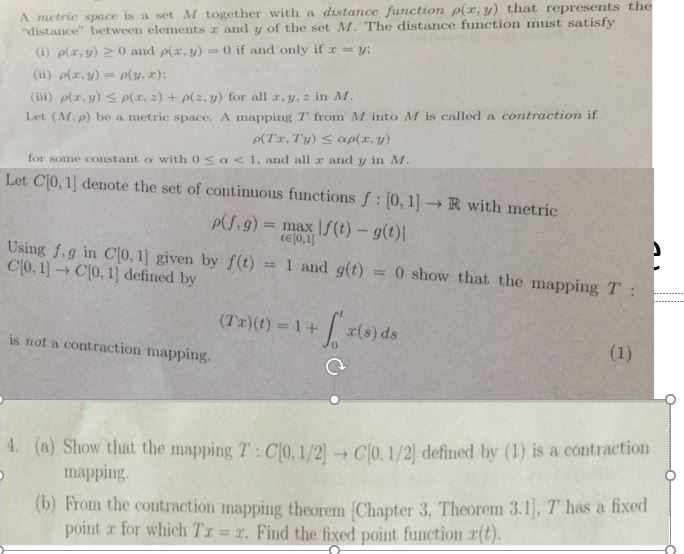# A metric space is a set M together with a distance function p(r, y) that represents the"distance" between elements x and y of the set A. The distance function must satisfy0 and p(z,y)0 if and only if xy;(i) ρ(z,y)(iii) y) a(z,z) + (s,y) for all z, y,s in M.Let (M.p) be a metric space. A mapping T from M into M is called a contraction iffor some constant o with o sac1, and all r and y in MLet C(0, 1] denote the set of continuous functions f 0,1]R with metricp(f.g) max If (t) - g(ttejo.lUsing f.g in Cl0. 1] given by fe) -1 and gt) 0 show that the mappingClo. 1] → C[0, 1] defined by(Ta)(t) 1+r(s) dsis not a contraction mapping.(a) Showy that the mapping T : С 0.1/2 -C 0 1/21 defined by (1) is a contraction(b) Prom the contraction mapping theorem Chapter 3, Theorem 3.1], T has a fixed4.mappingpoint x for which Tz # r. Find the fixed point function r(t)

Question
20 views

pls explain to me step by step. pls write clearly and dont skip any steps. thankshelp_outlineImage TranscriptioncloseA metric space is a set M together with a distance function p(r, y) that represents the "distance" between elements x and y of the set A. The distance function must satisfy 0 and p(z,y) 0 if and only if x y; (i) ρ(z,y) (iii) y) a(z,z) + (s,y) for all z, y,s in M. Let (M.p) be a metric space. A mapping T from M into M is called a contraction if for some constant o with o sac1, and all r and y in M Let C(0, 1] denote the set of continuous functions f 0,1]R with metric p(f.g) max If (t) - g(t tejo.l Using f.g in Cl0. 1] given by fe) -1 and gt) 0 show that the mapping Clo. 1] → C[0, 1] defined by (Ta)(t) 1+r(s) ds is not a contraction mapping. (a) Showy that the mapping T : С 0.1/2 -C 0 1/21 defined by (1) is a contraction (b) Prom the contraction mapping theorem Chapter 3, Theorem 3.1], T has a fixed 4. mapping point x for which Tz # r. Find the fixed point function r(t) fullscreen
check_circle

Step 1

To test the contraction mapping property for the same operator T in two different settings . (C[0,1] and C[0,1/2] )

Step 2

By definition, a contractive map T: X ->X has the property that it reduces distances between pairs of point in X. If T is a contraction mapping, the fixed point therorem says there is at least one fixed point for the mapping T. In other words, there exists a point x in T such that T(x)=x

Step 3

In the first part of the problem, we show that the mapT defined on C[0,1] with the supremum distance is NOT a contract...

### Want to see the full answer?

See Solution

#### Want to see this answer and more?

Solutions are written by subject experts who are available 24/7. Questions are typically answered within 1 hour.*

See Solution
*Response times may vary by subject and question.
Tagged in

### Math